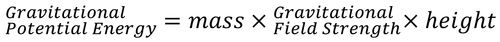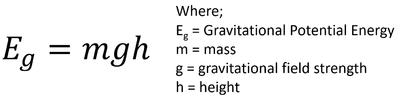# Gravitational Potential Energy Store

## Key Stage 3

### MeaningThe rock at the top of this stack has a large amount of energy in the gravitational potential energy store.

The gravitational potential energy store is the energy stored in an object that is in a gravitational field.

### About The Gravitational Potential Energy Store

The stronger the gravitational field the more energy in the gravitational potential energy store.
The greater the mass of the object the more energy in the gravitational potential energy store.
The greater the height of the object the more energy in the gravitational potential energy store.

### EquationThe equation for gravitational potential energy written in words.The equation for gravitational potential energy written in symbols.

### Calculating Gravitational Potential Energy

 A weight lifter lifts a 50kg mass a distance of 2.0m from the ground. Calculate the increase in gravitational potential energy of the mass. g on Earth is 9.8N/kg A pulley is used to lift a 12 tonne mass 0.80m above the ground. Calculate the change in energy in the gravitational potential store. g on Earth is 9.8N/kg Give your answer correct to two significant figures. During a rock slide a 320kg boulder falls from a height of 1450m to a height of 730m above sea level. Calculate the change in gravitational potential energy. g on Earth is 9.8N/kg Give your answer correct to two significant figures. 1. State the known quantities m = 50kg g = 9.8N/kg Δh = 2.0m 1. State the known quantities m = 12tonne = 12,000kg g = 9.8N/kg Δh = 0.80m 1. State the known quantities m = 320kg g = 9.8 N/kg Δh = h2 - h1 = 1450 - 730 = 720m 2. Substitute the numbers into the equation and solve. $$E_g = m g \Delta h$$ $$E_g = m \times g \times \Delta h$$ $$E_g = 50 \times 9.8 \times 2$$ $$E_g = 980J$$ 2. Substitute the numbers into the equation and solve. $$E_g = m g \Delta h$$ $$E_g = m \times g \times \Delta h$$ $$E_g = 0.80 \times 9.8 \times 12000$$ $$E_g = 94080J$$ $$E_g \approx 94000J$$ 2. Substitute the numbers into the equation and solve. $$E_g = m g \Delta h$$ $$E_g = m \times g \times \Delta h$$ $$E_g = 320 \times 9.8 \times 720$$ $$E_g = 2257920J$$ $$E_g \approx 2300000J$$

## Key Stage 4

### Meaning

The gravitational potential energy store is the energy stored in an object that is in a gravitational field.

Gravitational Potential Energy is a potential energy due to the position of a mass in a gravitational field.
The gravitational potential energy store of an object is related to three important factors:

### Equation

NB: You must memorise this equation!

Gravitational Potential Energy = (Mass) x (gravitational field strength) x (change in height)

$$E_g = m g \Delta h$$

Where:

Eg = Gravitational Potential Energy stored.

m = The mass of the object.

g = The gravitational field strength.

Δh = The change in height of the object.

### Calculating Change in Height from Gravitational Potential Energy

 An object of mass 5.0kg is lifted increasing its gravitational potential energy by 245J. Calculate the change in height of the object. Gravitational Field Strength on Earth is 9.8N/kg. A 3.6kg cat jumps vertically with an initial kinetic energy of 70J. Calculate the maximum height that the cat will be able to reach in this jump correct to two significant figures. Gravitational Field Strength on Earth is 9.8N/kg. A vacationing astronaut takes a bow and arrow to The Moon. The bow has an initial elastic potential energy store of 400J before releasing the arrow vertically. Given the mass of the arrow is 120g; calculate the maximum height that the arrow will reach before returning to the ground correct to two significant figures. Gravitational Field Strength on Earth is 1.6N/kg. 1. State the known quantities m = 5.0kg g = 9.8N/kg Eg = 245J 1. State the known quantities m = 3.6kg g = 9.8N/kg Eg = 70J (Since the law of Conservation of Energy states that energy is transferred from one store into another.) 1. State the known quantities m = 120g = 0.12kg g = 1.6N/kg Eg = 400J (Since the law of Conservation of Energy states that energy is transferred from one store into another.) 2. Substitute the numbers and evaluate. $$E_g = m g \Delta h$$ $$245 = 5 \times 9.8 \times h$$ $$245 = 49h$$ 2. Substitute the numbers and evaluate. $$E_g = m g \Delta h$$ $$70 = 3.6 \times 9.8 \times h$$ $$70 = 35.28h$$ 2. Substitute the numbers and evaluate. $$E_g = m g \Delta h$$ $$400 = 0.12 \times 1.6 \times h$$ $$400 = 0.192h$$ 3. Rearrange the equation and solve. $$h = \frac{245}{49}$$ $$h = 5m$$ 3. Rearrange the equation and solve. $$h = \frac{70}{35.28}$$ $$h = 1.984127m$$ $$h \approx 2.0m$$ 3. Rearrange the equation and solve. $$h = \frac{400}{0.192}$$ $$h = 2083.3m$$ $$h \approx 2100m$$ (The astronaut then dies because the arrow comes straight back down on them.)